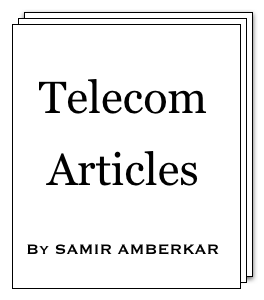online LTE test online C test Updated or New CDF explained5G Page5G Intent (a presentation)5G Intent (article)CV2X PageA Look at CV2X (a presentation)

 About Feedback Information Theory Modulation Multiple Access OSI Model Data Link layer SS7 Word about ATM GSM GPRS UMTS WiMAX LTE 5G Standard Reference Reference books Resources on Web Miscellaneous CV2X Mind Map Perl resources Magic MSC tool Bar graph tool C programming ASCII table Project Management Simple Google box HTML characters Site view counter 7 5 0 , 5 4 0 (May-2010 till Mar-2019)GMSK modulation

GSM GMSK modulation [Under GSM]

In this article, we would illustrate GSM GMSK modulation. Below are the equations specified in 3GPP TS 45.004:2.

Differential encoding is used; it means rather than every bit value, difference in consecutive bits is encoded as +1 or -1 (+1 is when bits are same, -1 otherwise), refer αi below. Along with equations, corresponding waveforms are shown for an example of one differential encoding of "+1".

Gaussian function with BT=0.3, T=48/13 μs

h(t) =
 1 δT . √ (2π)

e
 -t2 2δ2T2

 δ = ____ = 0.44168 with BT=0.3 √ln(2) 2πBTConvolution

 g(t) = h(t) * rect( t ) T

 rect( t ) = 1 if |t| < T T T 2

 rect( t ) = 0 if |t| > T T 2Integral

 . t' . G(t') = πh . ∫ g(u) du . -∞ .

 φ(t') = n . t'-iT . Σ ( αiπh . ∫ g(u) du ) i=1 . -∞ .

αi = { +1, -1 }
h = 0.5Phase shift of carrier

____
x(t') = (
 2Ec T
) . cos(2πf0t' + φ(t') + φ0)As you notice in above diagram, (modulation) phase shift increases and saturates after 2T. Above plots are drawn with gnuplot, more about it in later article.

In next article, we will take an interesting case of Frequency Correction Burst (FB) transmitted over FCCH.

References: GSM book by Mouly and Pautet.